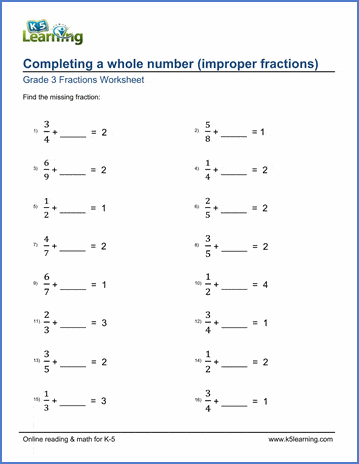# Completing whole numbers

## Fraction worksheets: completing a whole number

Below are six versions of our grade 3 math worksheet on completing a whole number with an improper fractions; students must find the improper fraction missing from the addition equation with whole number sum. These worksheets are pdf files.## More fractions worksheets

Explore all of our fractions worksheets, from dividing shapes into "equal parts" to multiplying and dividing improper fractions and mixed numbers.

## What is K5?

K5 Learning offers reading and math worksheets, workbooks and an online reading and math program for kids in kindergarten to grade 5.  We help your children build good study habits and excel in school.